Courses

# Test: Moment Of A Force - 2

## 20 Questions MCQ Test Engineering Mechanics | Test: Moment Of A Force - 2

Description
This mock test of Test: Moment Of A Force - 2 for Mechanical Engineering helps you for every Mechanical Engineering entrance exam. This contains 20 Multiple Choice Questions for Mechanical Engineering Test: Moment Of A Force - 2 (mcq) to study with solutions a complete question bank. The solved questions answers in this Test: Moment Of A Force - 2 quiz give you a good mix of easy questions and tough questions. Mechanical Engineering students definitely take this Test: Moment Of A Force - 2 exercise for a better result in the exam. You can find other Test: Moment Of A Force - 2 extra questions, long questions & short questions for Mechanical Engineering on EduRev as well by searching above.
QUESTION: 1

### Which of the following is correct in the determination of the moment direction by curling of wrist?

Solution:

The curled hand represents various thing. The direction of the moment axis is given by the thumb. The direction of the force is given by the fingers. As we place the fingers on the force and curl towards the rotational direction of the body about the axis.

QUESTION: 2

### Find the moment along R.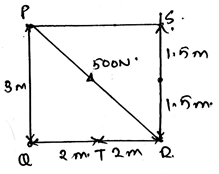Solution:

As we know that the moment is the cross product of the distance and the force we will try to apply the same here. But here we have number of forces components acting on the T point. So by adding all the moments caused by all the forces will give us the value as 0Nm.

QUESTION: 3

### For the below diagram determine the magnitude of the horizontal force applied at R which creates the same moment at P as by 75N.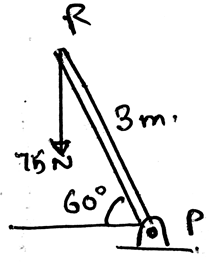Solution:

As we know that the moment is the cross product of the distance and the force we will try to apply the same here. We see that the perpendicular distance is 3sin60. Thus we get the distance. And hence multiply it with the force, moment = 112.5Nm. Because the force component perpendicular to the distance needs to be taken.

QUESTION: 4

Which of the following is true?

Solution:

When we consider about the dimensions we need to be careful.The moment is the vector quantity. Thus the value of the total moment caused by various forces acting on the body is the vector sum of all the vectors. Also the moments are not perpendicular to each other, unless it is specified.

QUESTION: 5

The moment axis is in the direction parallel to the plane of the force and the distance.

Solution:

The moment axis is always perpendicular to the planes of the force and the distance of the axis and the point of action of the force on the body. This means that the moment is the cross product of the force and the distance between the axis and the point of action of the force.

QUESTION: 6

Determine the resultant moment caused by the forces in vector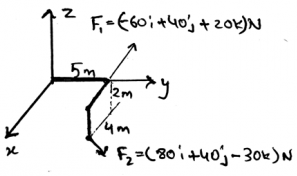Solution:

As we know that the moment is the cross product of the force and the distance between the point of contact of the force and the point about which moment needs to be calculated. Here distance r1 =5j m and r2 = 4i + 5j – 2k m. Thus doing the cross product will give the answer.

QUESTION: 7

Which of the following is correct w.r.t the moment (M) of the force (F) acting on the body at a distance L from the axis of the rotation?

Solution:

The moment of the force about the axis of rotation by the application of the force on the body is given by the cross product of both. If the force not perpendicular to the axis, and making angle θ then cosine form of angle is used. As usually used in the cross product.

QUESTION: 8

What does FLsinθ means/represents for the moment (M) of the force (F) acting on the body at a distance L from the axis of the rotation?

Solution:

The moment of the force about the axis of rotation by the application of the force on the body is given by the cross product of both. If the force not perpendicular to the axis, and making angle θ then cosine form of angle is used. Thus, FLsinθ represents the magnitude of the moment.

QUESTION: 9

The basic way of getting the direction of the moment caused by the force is:

Solution:

The basic way of doing so is to use right hand rule and not the left hand rule. The direction of the moment axis is given by the thumb. The direction of the force is given by the fingers. As we place the fingers on the force and curl towards the rotational direction of the body about the axis.

QUESTION: 10

If any force is applied in the direction of the positive x-axis, and there are three different point on which the moment of this force is to be calculated. Then if these three points are on the positive side of the y-axis with varying distance, then what will be the direction of the moment caused by the force to the individual point?

Solution:

If you will apply he right hand rule on the system given then the right answer is the positive z-axis. Which is because the force is lying on the x-axis and is heading towards the positive infinity of the x-axis. And the points are in the positive y-axis. Apply the rule, and get the direction.

QUESTION: 11

If a force applied at any point in its line of action and is still creating the same moment about any fixed point say P, then the force is said to be______________

Solution:

If a force applied at any point in its line of action and is still creating the same moment about any fixed point say P, then the force is said to be sliding vector. This is because the moment of the force which is acting on its line of axis at the point P is same throughout. Whatever be the direction of the distance.

QUESTION: 12

If a force applied at any point in its line of action and is still creating the same moment about any fixed point say P, then the force is said to be sliding vector. What is the name of this property?

Solution:

If a force applied at any point in its line of action and is still creating the same moment about any fixed point say P, then the force is said to be sliding vector. This is because the moment of the force which is acting on its line of axis at the point P is same throughout. This is known as the principle transmissibility of the force.

QUESTION: 13

We can express the force in the Cartesian form.

Solution:

Yes, we can prepare the moment in the Cartesian form. As the moment in the 3D is the vector. Which can be easily made in the form of Cartesian coordinates. Also it can be seen that the moment is the cross product of the force and the distance, hence the moment is in vector form.

QUESTION: 14

M = ∑(rxF) represents what?

Solution:

The given equation represents the total moment of the forces which are acting on the body. That is the summation of all the rxF. Where the r is the distance of the axis from the point of action of the force on the body. And thus this is the total summation of the moments of all the forces acting on the body.

QUESTION: 15

If a 12m high tree is being pulled by the tractor, by a rope tied over the top. With the tractor at a linear distance of 12m and 4m away perpendicularly from the tree. If the force applied by the tractor is 2KN then what is the moment caused about the roots of the tree?

Solution:

The force developed is 2KN, and the roots are having the coordinate (0, 0, 0). Coordinates of the top of the tree is (0, 0, 12). The tractor’s coordinates are (4, 12, 0). Thus applying the cross product on the force and the distance of the tractor from the roots we get the answer as -16.5i+5.51j KNm.

QUESTION: 16

If F = F1+F2, then moment of this force F about a point at a distance r is M=rxF1 + rxF2.

Solution:

If F = F1+F2, then moment of this force F about a point at a distance r is M=rxF1 + rxF2.
As M = rx(F) = rx(F1 + F2) = rxF1 + rxF2. This is known as the principle of moments. As the force is a vector quantity thus this is the distributive property which we apply to get the answer.

QUESTION: 17

Determine the moment of the force about the point X.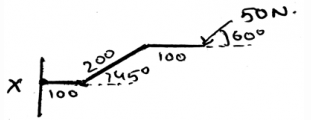Solution:

As we know that the moment is the cross product of the force and the distance between the point of contact of the force and the point about which moment needs to be calculated. Thus forming the distance vector and then crossing it with the force will give us the answer. Remember force also needs to be in the vector form for doing the cross product.

QUESTION: 18

Determine the moment about the point Q by the force shown as 400N.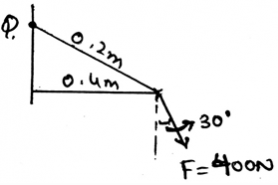Solution:

As we know that the moment is the cross product of the force and the distance between the point of contact of the force and the point about which moment needs to be calculated. Thus forming the distance vector and then crossing it with the force will give us the answer. Remember force also needs to be in the vector form for doing the cross product.

QUESTION: 19

Determine the moment about the point P.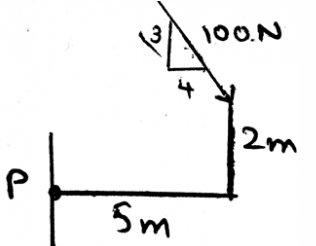Solution:

As we know that the moment is the cross product of the force and the distance between the point of contact of the force and the point about which moment needs to be calculated. Thus forming the distance vector and then crossing it with the force will give us the answer. Remember force also needs to be in the vector form for doing the cross product.

QUESTION: 20

Determine the magnitude of the resultant moment caused by the forces.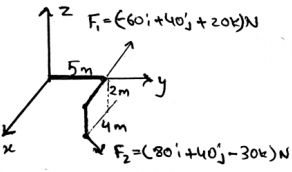Solution:

As we know that the moment is the cross product of the force and the distance between the point of contact of the force and the point about which moment needs to be calculated. Here distance r1 =5j m and r2 = 4i + 5j – 2k m. Thus doing the cross product will give the answer.